• 1.GraphSAGE 本文代码源于 DGL 的 Example 的，感兴趣可以去 github 上面查看。 阅读代码的本意是加深对论文的理解，其次是看下大佬们实现算法的一些方式方法。当然，在阅读 GraphSAGE 代码时我也发现了之前忽视的 ...
1.GraphSAGE
本文代码源于 DGL 的 Example 的，感兴趣可以去 github 上面查看。
阅读代码的本意是加深对论文的理解，其次是看下大佬们实现算法的一些方式方法。当然，在阅读 GraphSAGE 代码时我也发现了之前忽视的 GraphSAGE 的细节问题和一些理解错误。比如说：之前忽视了 GraphSAGE 的四种聚合方式的具体实现，对 Alogrithm 2 的算法理解也有问题，再回头看那篇 GraphSAGE 的推文时，实在惨不忍睹= =。
进入正题，简单回顾一下 GraphSAGE。
核心算法：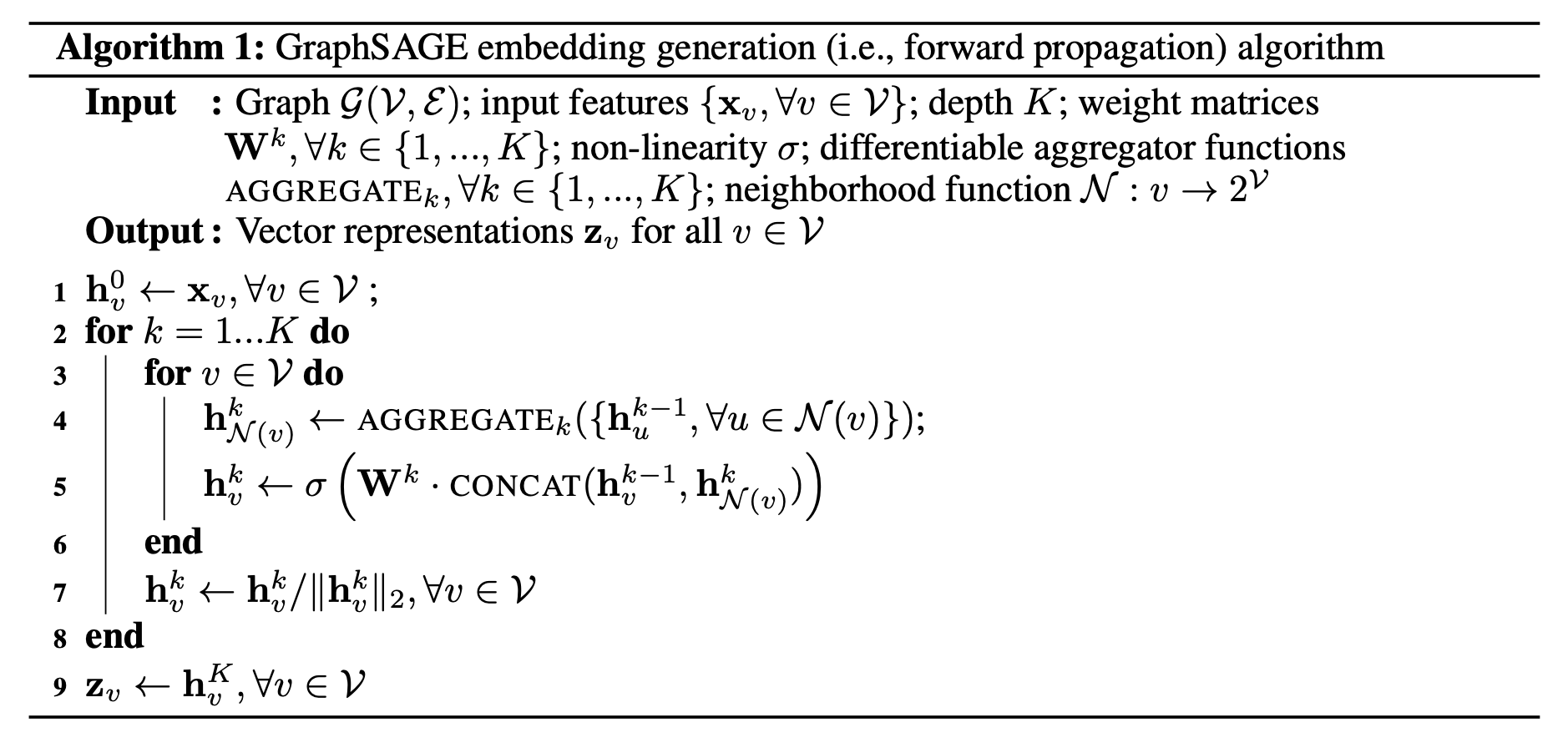2.SAGEConv
dgl 已经实现了 SAGEConv 层，所以我们可以直接导入。
有了 SAGEConv 层后，GraphSAGE 实现起来就比较简单。
和基于 GraphConv 实现 GCN 的唯一区别在于把 GraphConv 改成了 SAGEConv：
class GraphSAGE(nn.Module):
def __init__(self,
g,
in_feats,
n_hidden,
n_classes,
n_layers,
activation,
dropout,
aggregator_type):
super(GraphSAGE, self).__init__()
self.layers = nn.ModuleList()
self.g = g
# input layer
self.layers.append(SAGEConv(in_feats, n_hidden, aggregator_type,
feat_drop=dropout, activation=activation))
# hidden layers
for i in range(n_layers - 1):
self.layers.append(SAGEConv(n_hidden, n_hidden, aggregator_type,
feat_drop=dropout, activation=activation))
# output layer
self.layers.append(SAGEConv(n_hidden, n_classes, aggregator_type,
feat_drop=dropout, activation=None)) # activation None

def forward(self, features):
h = features
for layer in self.layers:
h = layer(self.g, h)
return h

来看一下 SAGEConv 是如何实现的
SAGEConv 接收七个参数：
in_feat：输入的特征大小，可以是一个整型数，也可以是两个整型数。如果用在单向二部图上，则可以用整型数来分别表示源节点和目的节点的特征大小，如果只给一个的话，则默认源节点和目的节点的特征大小一致。需要注意的是，如果参数 aggregator_type 为 gcn 的话，则源节点和目的节点的特征大小必须一致；out_feats：输出特征大小；aggregator_type：聚合类型，目前支持 mean、gcn、pool、lstm，比论文多一个 gcn 聚合，gcn 聚合可以理解为周围所有的邻居结合和当前节点的均值；feat_drop=0.：特征 drop 的概率，默认为 0；bias=True：输出层的 bias，默认为 True；norm=None：归一化，可以选择一个归一化的方式，默认为 Noneactivation=None：激活函数，可以选择一个激活函数去更新节点特征，默认为 None。
class SAGEConv(nn.Module):

def __init__(self,
in_feats,
out_feats,
aggregator_type,
feat_drop=0.,
bias=True,
norm=None,
activation=None):
super(SAGEConv, self).__init__()

# expand_as_pair 函数可以返回一个二维元组。
self._in_src_feats, self._in_dst_feats = expand_as_pair(in_feats)
self._out_feats = out_feats
self._aggre_type = aggregator_type
self.norm = norm
self.feat_drop = nn.Dropout(feat_drop)
self.activation = activation
# aggregator type: mean/pool/lstm/gcn
if aggregator_type == 'pool':
self.fc_pool = nn.Linear(self._in_src_feats, self._in_src_feats)
if aggregator_type == 'lstm':
self.lstm = nn.LSTM(self._in_src_feats, self._in_src_feats, batch_first=True)
if aggregator_type != 'gcn':
self.fc_self = nn.Linear(self._in_dst_feats, out_feats, bias=bias)
self.fc_neigh = nn.Linear(self._in_src_feats, out_feats, bias=bias)
self.reset_parameters()

def reset_parameters(self):
"""初始化参数
这里的 gain 可以从 calculate_gain 中获取针对非线形激活函数的建议的值
用于初始化参数
"""
gain = nn.init.calculate_gain('relu')
if self._aggre_type == 'pool':
nn.init.xavier_uniform_(self.fc_pool.weight, gain=gain)
if self._aggre_type == 'lstm':
self.lstm.reset_parameters()
if self._aggre_type != 'gcn':
nn.init.xavier_uniform_(self.fc_self.weight, gain=gain)
nn.init.xavier_uniform_(self.fc_neigh.weight, gain=gain)

def _lstm_reducer(self, nodes):
"""LSTM reducer
NOTE(zihao): lstm reducer with default schedule (degree bucketing)
is slow, we could accelerate this with degree padding in the future.
"""
m = nodes.mailbox['m'] # (B, L, D)
batch_size = m.shape
h = (m.new_zeros((1, batch_size, self._in_src_feats)),
m.new_zeros((1, batch_size, self._in_src_feats)))
_, (rst, _) = self.lstm(m, h)
return {'neigh': rst.squeeze(0)}

def forward(self, graph, feat):
""" SAGE 层的前向传播
接收 DGLGraph 和 Tensor 格式的节点特征
"""
# local_var 会返回一个作用在内部函数中使用的 Graph 对象
# 外部数据的变化不会影响到这个 Graph
# 可以理解为保护数据不被意外修改
graph = graph.local_var()

if isinstance(feat, tuple):
feat_src = self.feat_drop(feat)
feat_dst = self.feat_drop(feat)
else:
feat_src = feat_dst = self.feat_drop(feat)

h_self = feat_dst

# 根据不同的聚合类型选择不同的聚合方式
# 值得注意的是，论文在 3.3 节只给出了三种聚合方式
# 而这里却多出来一个 gcn 聚合
# 具体原因将在后文给出
if self._aggre_type == 'mean':
graph.srcdata['h'] = feat_src
graph.update_all(fn.copy_src('h', 'm'), fn.mean('m', 'neigh'))
h_neigh = graph.dstdata['neigh']
elif self._aggre_type == 'gcn':
# check_eq_shape 用于检查源节点和目的节点的特征大小是否一致
check_eq_shape(feat)
graph.srcdata['h'] = feat_src
graph.dstdata['h'] = feat_dst     # same as above if homogeneous
graph.update_all(fn.copy_src('h', 'm'), fn.sum('m', 'neigh'))
# divide in_degrees
degs = graph.in_degrees().to(feat_dst)
h_neigh = (graph.dstdata['neigh'] + graph.dstdata['h']) / (degs.unsqueeze(-1) + 1)
elif self._aggre_type == 'pool':
graph.srcdata['h'] = F.relu(self.fc_pool(feat_src))
graph.update_all(fn.copy_src('h', 'm'), fn.max('m', 'neigh'))
h_neigh = graph.dstdata['neigh']
elif self._aggre_type == 'lstm':
graph.srcdata['h'] = feat_src
graph.update_all(fn.copy_src('h', 'm'), self._lstm_reducer)
h_neigh = graph.dstdata['neigh']
else:
raise KeyError('Aggregator type {} not recognized.'.format(self._aggre_type))

# GraphSAGE GCN does not require fc_self.
if self._aggre_type == 'gcn':
rst = self.fc_neigh(h_neigh)
else:
rst = self.fc_self(h_self) + self.fc_neigh(h_neigh)
# activation
if self.activation is not None:
rst = self.activation(rst)
# normalization
if self.norm is not None:
rst = self.norm(rst)
return rst


reset_parameters 函数那里有一个 gain，初始化参数服从 Xavier 均匀分布：

W

∼

U

[

−

gain

6

n

j

+

n

j

+

1

,

gain

gain

6

n

j

+

n

j

+

1

]

W \sim U[- \frac{\text{gain} \sqrt{6}}{\sqrt{n_j+n_{j+1}}}, \text{gain} \frac{\text{gain} \sqrt{6}}{\sqrt{n_j+n_{j+1}}}] \\

仔细阅读论文时会发现，在实验部分作者给出了四种方式的聚合方法：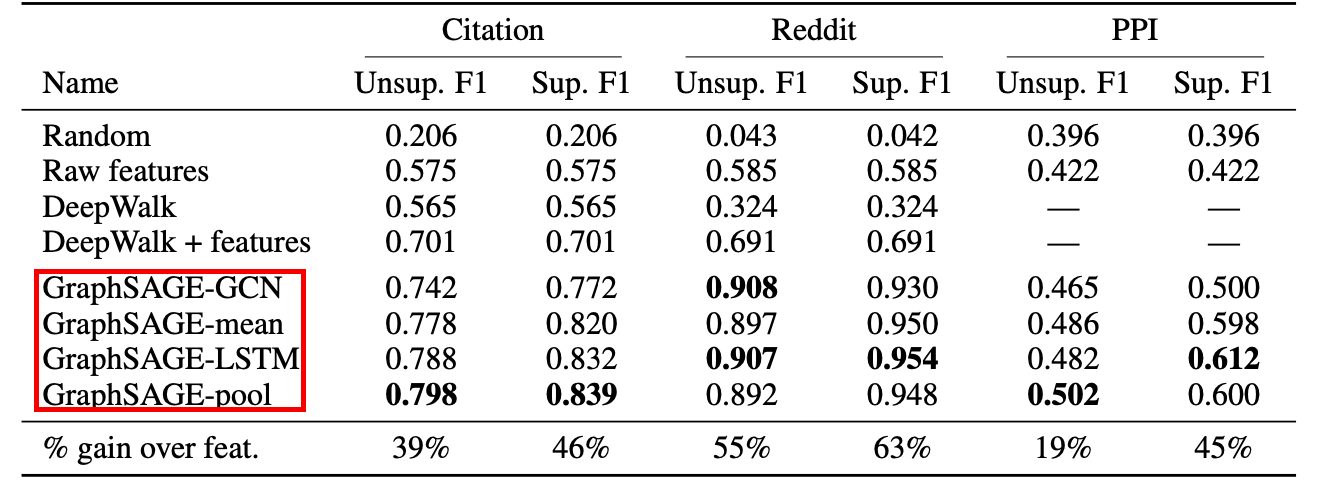配合着论文，我们来阅读下代码
MEAN 聚合器：首先对邻居节点进行均值聚合，然后当前节点特征与邻居节点特征该分别送入全连接网络后相加得到结果，对应伪代码如下：

h

N

(

v

)

k

←

MEAN

k

(

{

h

u

k

−

1

,

∀

u

∈

N

(

v

)

}

)

h

v

k

←

σ

(

W

k

⋅

CONCAT

(

{

h

v

k

−

1

,

h

N

(

v

)

k

}

)

h_{N(v)}^k \leftarrow \text{MEAN}_k(\{ \mathbf{h}_u^{k-1}, \forall u \in N(v )\}) \\ h_v^k \leftarrow \sigma(\mathbf{W^k} \cdot \text{CONCAT}(\{\mathbf{h}_v^{k-1}, h_{N(v)}^k\} ) \\

对应代码如下：
h_self = feat_dst
graph.srcdata['h'] = feat_src
graph.update_all(fn.copy_src('h', 'm'), fn.mean('m', 'neigh'))
h_neigh = graph.dstdata['neigh']
# 公式里写的是 concat，这里是 element-wise 的和。
# 稍微有些出入，不过问题不大。
rst = self.fc_self(h_self) + self.fc_neigh(h_neigh)

GCN 聚合：首先对邻居节点的特征和自身节点的特征求均值，得到的聚合特征送入到全连接网络中，对应论文公式如下：

h

v

k

←

σ

(

W

⋅

MEAN

(

{

h

v

k

−

1

}

∪

h

u

k

−

1

,

∀

u

∈

N

(

v

)

}

)

h_v^k \leftarrow \sigma(\mathbf{W} \cdot \text{MEAN}(\{\mathbf{h}_v^{k-1}\} \cup \mathbf{h}_u^{k-1}, \forall u \in N(v )\} )

对应代码如下：
graph.srcdata['h'] = feat_src
graph.dstdata['h'] = feat_dst
graph.update_all(fn.copy_src('h', 'm'), fn.sum('m', 'neigh'))
# divide in_degrees
degs = graph.in_degrees().to(feat_dst)
# 公式中给出集合并集，这里做 element-wise 的和，问题也不大。
h_neigh = (graph.dstdata['neigh'] + graph.dstdata['h']) / (degs.unsqueeze(-1) + 1)
rst = self.fc_neigh(h_neigh)

gcn 与 mean 的关键区别在于节点邻居节点和当前节点取平均的方式：gcn 是直接将当前节点和邻居节点取平均，而 mean 聚合是 concat 当前节点的特征和邻居节点的特征，所以前者只经过一个全连接层，而后者是分别经过全连接层。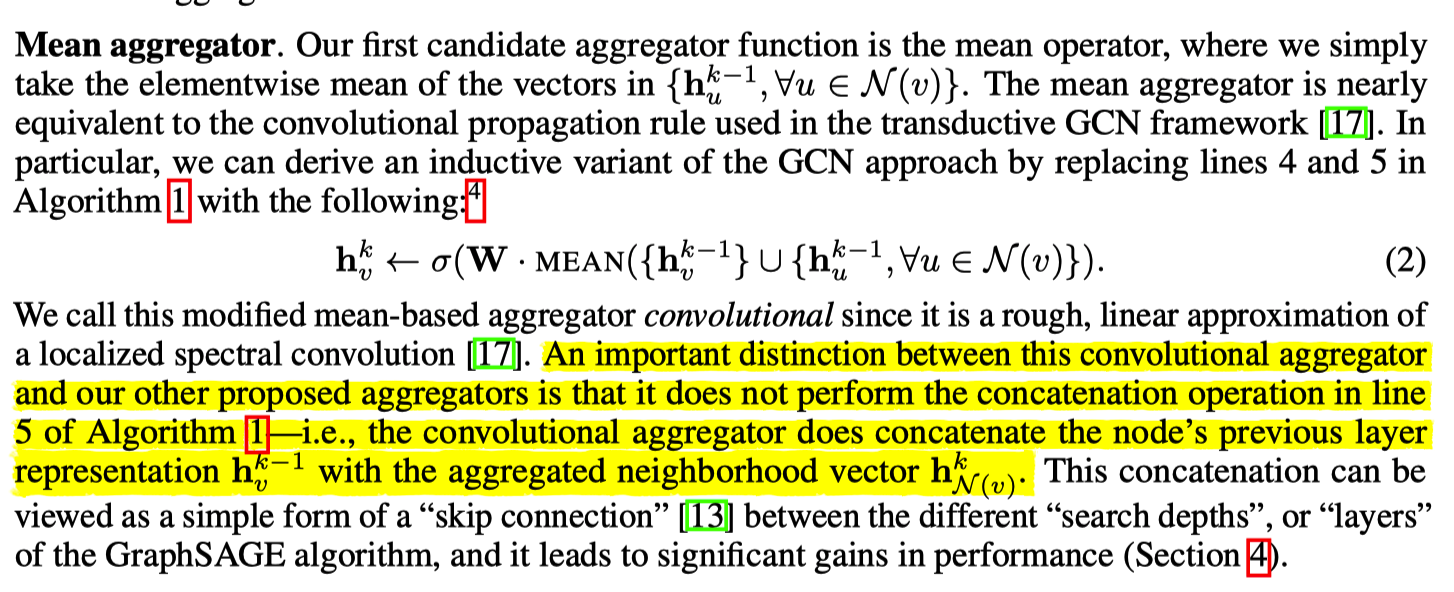这里利用下斯坦福大学的同学实现的 GCN 聚合器的解释，如果不明白的话，可以去其 github 仓库查看源码：
class MeanAggregator(Layer):
"""
Aggregates via mean followed by matmul and non-linearity.
"""

class GCNAggregator(Layer):
"""
Aggregates via mean followed by matmul and non-linearity.
Same matmul parameters are used self vector and neighbor vectors.
"""

POOL 聚合器：池化方法中，每一个节点的向量都会对应一个全连接神经网络，然后基于 elementwise 取最大池化操作。对应公式如下：

AGGREGATE

k

p

o

o

l

=

max

(

{

W

p

o

o

l

h

u

i

k

+

b

,

∀

u

i

∈

N

(

v

)

}

)

\text{AGGREGATE}_k^{pool} = \text{max}( \{\mathbf{W}_{pool} \mathbf{h}_{u_i}^k + \mathbf{b}, \forall u_i \in N(v) \} ) \\

对应代码如下：
graph.srcdata['h'] = F.relu(self.fc_pool(feat_src))
graph.update_all(fn.copy_src('h', 'm'), fn.max('m', 'neigh'))
h_neigh = graph.dstdata['neigh']

LSTM 聚合器：其表达能力比 mean 聚合器要强，但是 LSTM 是非对称的，即其考虑节点的顺序性，论文作者通过将节点进行随机排列来调整 LSTM 对无序集的支持。
def _lstm_reducer(self, nodes):
"""LSTM reducer
"""
m = nodes.mailbox['m'] # (B, L, D)
batch_size = m.shape
h = (m.new_zeros((1, batch_size, self._in_src_feats)),
m.new_zeros((1, batch_size, self._in_src_feats)))
_, (rst, _) = self.lstm(m, h)
return {'neigh': rst.squeeze(0)}

graph.srcdata['h'] = feat_src
graph.update_all(fn.copy_src('h', 'm'), self._lstm_reducer)
h_neigh = graph.dstdata['neigh']

以上便是利用 SAGEConv 实现 GraphSAGE 的方法，剩余训练的内容与前文介绍 GCN 一致，不再进行介绍。
3.Neighbor sampler
这里再介绍一种基于节点邻居采样并利用 minibatch 的方法进行前向传播的实现。
这种方法适用于大图，并且能够并行计算。
import dgl
import numpy as np
import torch as th
import torch.nn as nn
import torch.nn.functional as F
import torch.optim as optim
from dgl.nn import SAGEConv
import time
from dgl.data import RedditDataset
import tqdm

首先是邻居采样（NeighborSampler），这个最好配合着 PinSAGE 的实现来看：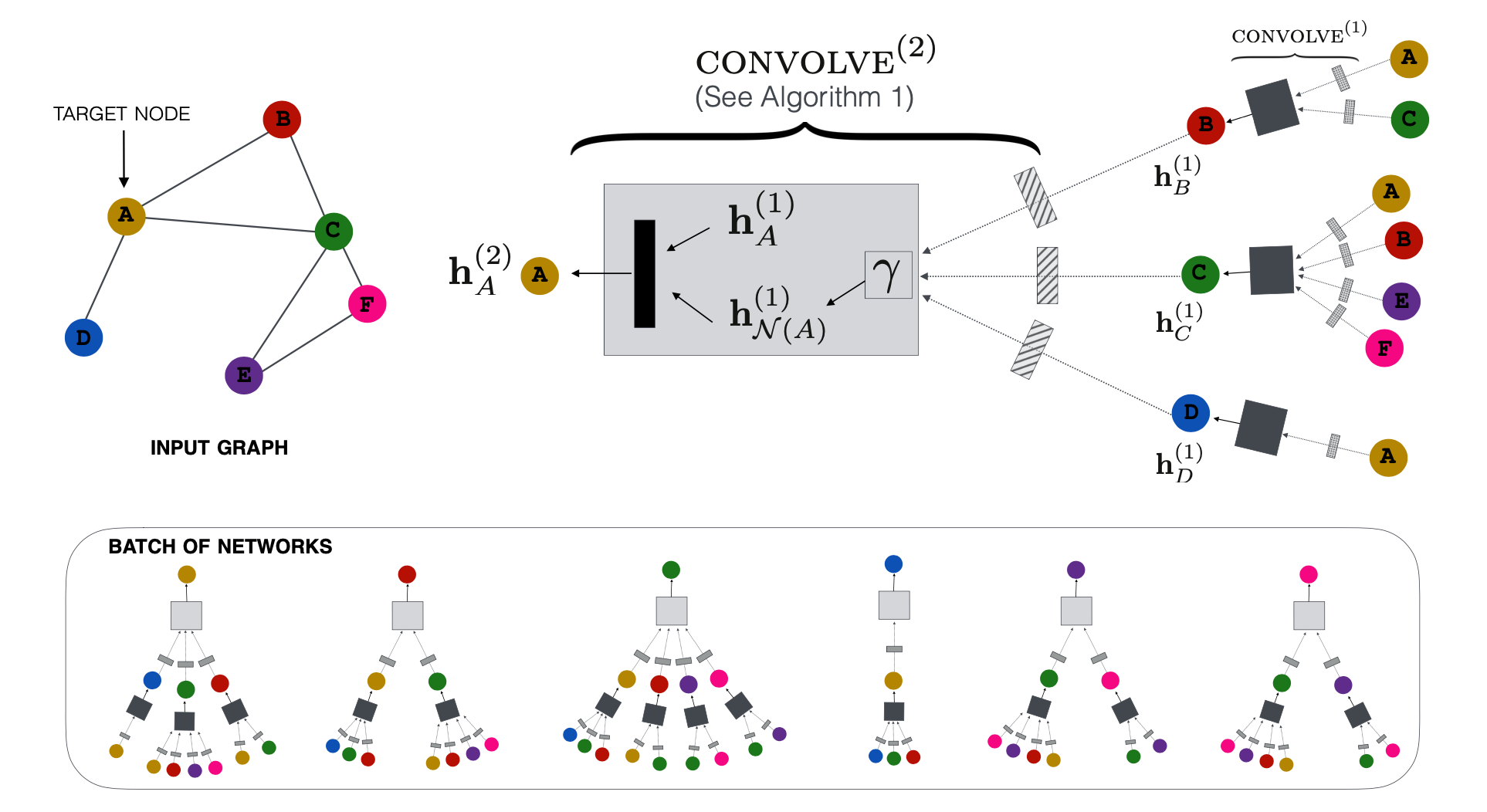我们关注下上半部分，首先对节点 A 的一阶邻居进行采样，然后再进行二阶邻居采样，节点 A 的二阶邻居可能会包括节点 A 及其一阶邻居。
Neighbor Sampler 函数的实现目的与之类似，首先获取最右边的种子节点，然后依次进行一阶采样和二阶采样。采样的方向是从左到右，而特征聚合方向是从从右到左。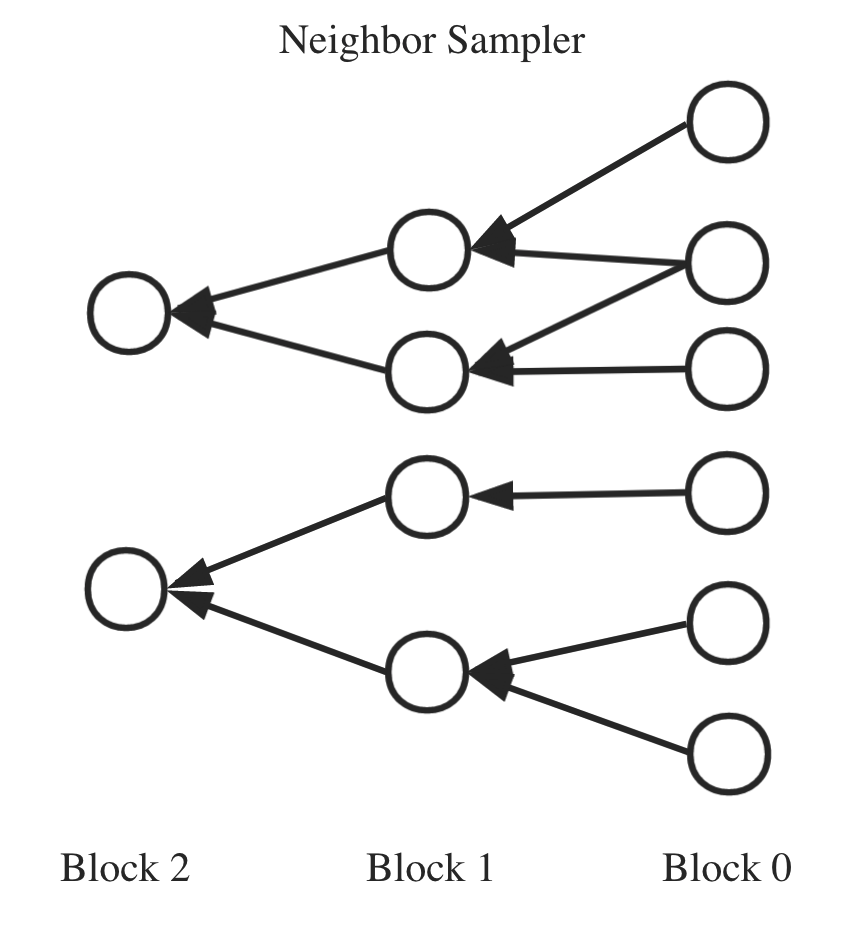class NeighborSampler(object):
def __init__(self, g, fanouts):
"""
g 为 DGLGraph；
fanouts 为采样节点的数量，实验使用 10,25，指一阶邻居采样 10 个，二阶邻居采样 25 个。
"""
self.g = g
self.fanouts = fanouts

def sample_blocks(self, seeds):
seeds = th.LongTensor(np.asarray(seeds))
blocks = []
for fanout in self.fanouts:
# sample_neighbors 可以对每一个种子的节点进行邻居采样并返回相应的子图
# replace=True 表示用采样后的邻居节点代替所有邻居节点
frontier = dgl.sampling.sample_neighbors(g, seeds, fanout, replace=True)
# 将图转变为可以用于消息传递的二部图（源节点和目的节点）
# 其中源节点的 id 也可能包含目的节点的 id（原因上面说了）
# 转变为二部图主要是为了方便进行消息传递
block = dgl.to_block(frontier, seeds)
# 获取新图的源节点作为种子节点，为下一层作准备
# 之所以是从 src 中获取种子节点，是因为采样操作相对于聚合操作来说是一个逆向操作
seeds = block.srcdata[dgl.NID]
# 把这一层放在最前面。
# PS：如果数据量大的话，插入操作是不是不太友好。
blocks.insert(0, block)
return blocks

Algorithm 2 伪代码如下所示，NeighborSampler 对应 Algorithm 2 算法的 1-7 步：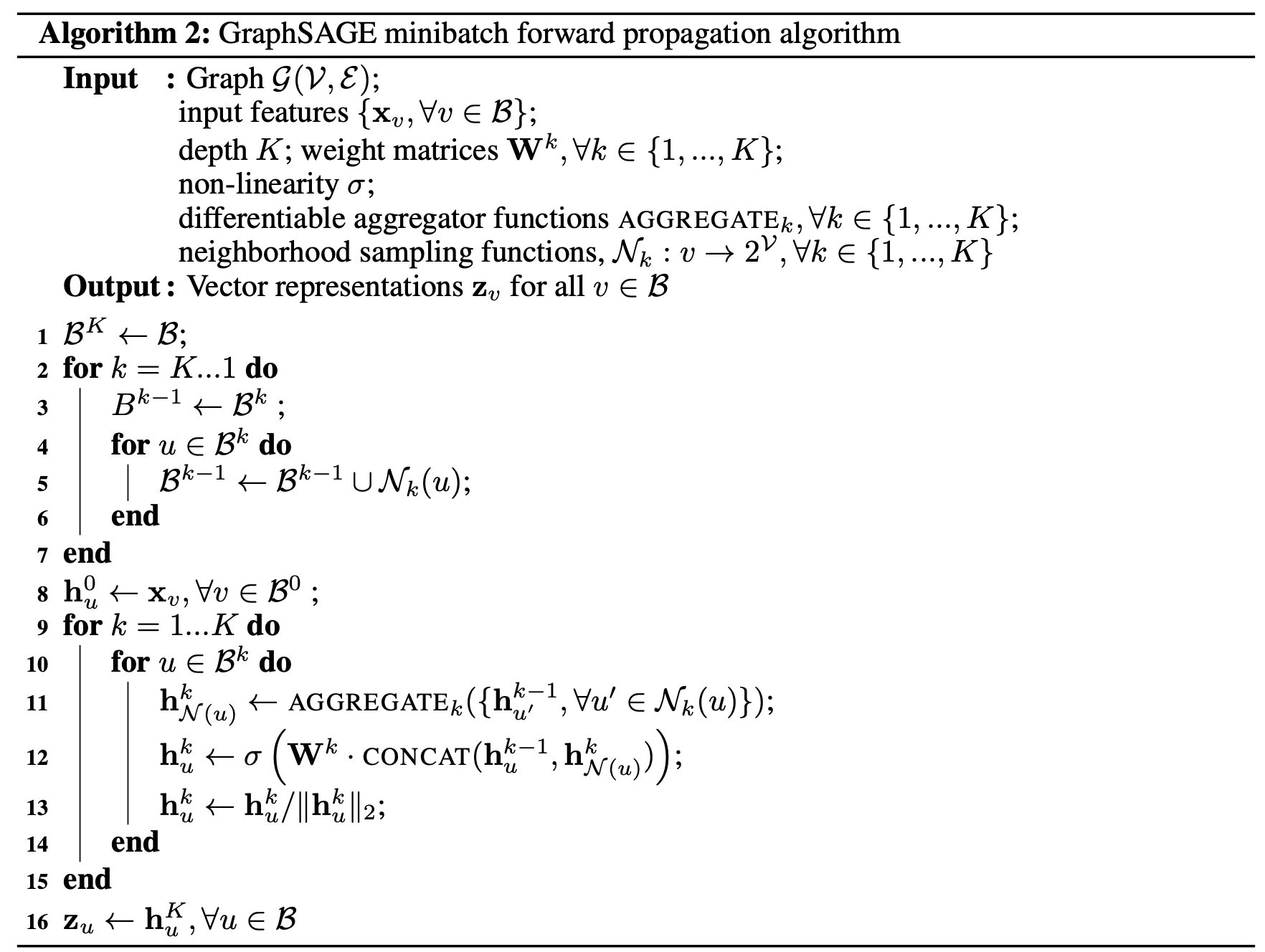# GraphSAGE 的代码实现
class GraphSAGE(nn.Module):
def __init__(self,
in_feats,
n_hidden,
n_classes,
n_layers,
activation,
dropout):
super().__init__()
self.n_layers = n_layers
self.n_hidden = n_hidden
self.n_classes = n_classes
self.layers = nn.ModuleList()
self.layers.append(SAGEConv(in_feats, n_hidden, 'mean'))
for i in range(1, n_layers - 1):
self.layers.append(dglnn.SAGEConv(n_hidden, n_hidden, 'mean'))
self.layers.append(SAGEConv(n_hidden, n_classes, 'mean'))
self.dropout = nn.Dropout(dropout)
self.activation = activation

def forward(self, blocks, x):
# block 是我们采样获得的二部图，这里用于消息传播
# x 为节点特征
h = x
for l, (layer, block) in enumerate(zip(self.layers, blocks)):
h_dst = h[:block.number_of_dst_nodes()]
h = layer(block, (h, h_dst))
if l != len(self.layers) - 1:
h = self.activation(h)
h = self.dropout(h)
return h

def inference(self, g, x, batch_size, device):
# inference 用于评估测试，针对的是完全图
# 目前会出现重复计算的问题，优化方案还在 to do list 上
nodes = th.arange(g.number_of_nodes())
for l, layer in enumerate(self.layers):
y = th.zeros(g.number_of_nodes(),
self.n_hidden if l != len(self.layers) - 1 else self.n_classes)
for start in tqdm.trange(0, len(nodes), batch_size):
end = start + batch_size
batch_nodes = nodes[start:end]
block = dgl.to_block(dgl.in_subgraph(g, batch_nodes), batch_nodes)
input_nodes = block.srcdata[dgl.NID]
h = x[input_nodes].to(device)
h_dst = h[:block.number_of_dst_nodes()]
h = layer(block, (h, h_dst))
if l != len(self.layers) - 1:
h = self.activation(h)
h = self.dropout(h)
y[start:end] = h.cpu()
x = y
return y

def compute_acc(pred, labels):
"""
计算准确率
"""
return (th.argmax(pred, dim=1) == labels).float().sum() / len(pred)

def evaluate(model, g, inputs, labels, val_mask, batch_size, device):
"""
评估模型，调用 model 的 inference 函数
"""
model.eval()
pred = model.inference(g, inputs, batch_size, device)
model.train()

def load_subtensor(g, labels, seeds, input_nodes, device):
"""
将一组节点的特征和标签复制到 GPU 上。
"""
batch_inputs = g.ndata['features'][input_nodes].to(device)
batch_labels = labels[seeds].to(device)
return batch_inputs, batch_labels

# 参数设置
gpu = -1
num_epochs = 20
num_hidden = 16
num_layers = 2
fan_out = '10,25'
batch_size = 1000
log_every = 20  # 记录日志的频率
eval_every = 5
lr = 0.003
dropout = 0.5
num_workers = 0  # 用于采样进程的数量

if gpu >= 0:
device = th.device('cuda:%d' % gpu)
else:
device = th.device('cpu')

# NumNodes: 232965
# NumEdges: 114848857
# NumFeats: 602
# NumClasses: 41
# NumTrainingSamples: 153431
# NumValidationSamples: 23831
# NumTestSamples: 55703
data = RedditDataset(self_loop=True)
features = th.Tensor(data.features)
in_feats = features.shape
labels = th.LongTensor(data.labels)
n_classes = data.num_labels
# Construct graph
g = dgl.graph(data.graph.all_edges())
g.ndata['features'] = features

开始训练：
train_nid = th.LongTensor(np.nonzero(train_mask))

# Create sampler
sampler = NeighborSampler(g, [int(fanout) for fanout in fan_out.split(',')])

# Create PyTorch DataLoader for constructing blocks
# collate_fn 参数指定了 sampler，可以对 batch 中的节点进行采样
dataset=train_nid.numpy(),
batch_size=batch_size,
collate_fn=sampler.sample_blocks,
shuffle=True,
drop_last=False,
num_workers=num_workers)

# Define model and optimizer
model = GraphSAGE(in_feats, num_hidden, n_classes, num_layers, F.relu, dropout)
model = model.to(device)
loss_fcn = nn.CrossEntropyLoss()
loss_fcn = loss_fcn.to(device)

# Training loop
avg = 0
iter_tput = []
for epoch in range(num_epochs):
tic = time.time()

tic_step = time.time()

input_nodes = blocks.srcdata[dgl.NID]
seeds = blocks[-1].dstdata[dgl.NID]

# Load the input features as well as output labels
batch_inputs, batch_labels = load_subtensor(g, labels, seeds, input_nodes, device)

# Compute loss and prediction
batch_pred = model(blocks, batch_inputs)
loss = loss_fcn(batch_pred, batch_labels)
loss.backward()
optimizer.step()

iter_tput.append(len(seeds) / (time.time() - tic_step))
if step % log_every == 0:
acc = compute_acc(batch_pred, batch_labels)
gpu_mem_alloc = th.cuda.max_memory_allocated() / 1000000 if th.cuda.is_available() else 0
print('Epoch {:05d} | Step {:05d} | Loss {:.4f} | Train Acc {:.4f} | Speed (samples/sec) {:.4f} | GPU {:.1f} MiB'.format(
epoch, step, loss.item(), acc.item(), np.mean(iter_tput[3:]), gpu_mem_alloc))

toc = time.time()
print('Epoch Time(s): {:.4f}'.format(toc - tic))
if epoch >= 5:
avg += toc - tic
if epoch % eval_every == 0 and epoch != 0:
eval_acc = evaluate(model, g, g.ndata['features'], labels, val_mask, batch_size, device)
print('Eval Acc {:.4f}'.format(eval_acc))

print('Avg epoch time: {}'.format(avg / (epoch - 4)))


4.Reference
Github:dmlc/dglwilliamleif/GraphSAGE
关注公众号跟踪最新内容：阿泽的学习笔记。展开全文GNN 图神经网络 图卷积神经网络 深度学习
• 最近在尝试使用 GraphSAGE 做 embedding，做个笔记。 G 图结构 文件：toy-ppi-G.json 数据通过 json 加载之后，得到一个 dict，其中包含的关键字：[‘nodes’, ‘directed’, ‘multigraph’, ‘graph’, ‘links’]...
最近在尝试使用 GraphSAGE 做 embedding，做个笔记。
G 图结构
文件：toy-ppi-G.json
数据通过 json 加载之后，得到一个 dict，其中包含的关键字：[‘nodes’, ‘directed’, ‘multigraph’, ‘graph’, ‘links’]。
nodes
G_data[‘nodes’] 包含所有的节点, 每个节点（node）包含属性 [‘test’, ‘label’, ‘id’, ‘val’, ‘feature’]。
node
test 和 val 是 bool 值。同一个结点，test 和 val 不同时为 True。 id 就是结点的 id 喽。 feature 是个 list，大小是 50，其中的元素是 float。 label 也是个 list，大小是 121, 类型是 int。注意 label 不是 one-hot。（为什么是 121 维呢？）
directed
bool
multigraph
bool
graph
dict 但是只有一个值 {name: “disjoint_union( , )”}
是一个 list ，每个元素是一个字典，包括 [‘source’, ‘target’, ‘test_removed’, ‘train_removed’]
所有 test_removed == True 的边的两端结点，都是 test == True 所有 train_removed == True 的边包括 test_removed == True 的边，以及两端 val == True 的边。
id map
dict id 到 id 的映射
class map
id 到 label 的映射。这个和 G[‘nodes’] 里面的 id 和 label 是一样的。
GraphSAGE 输入结构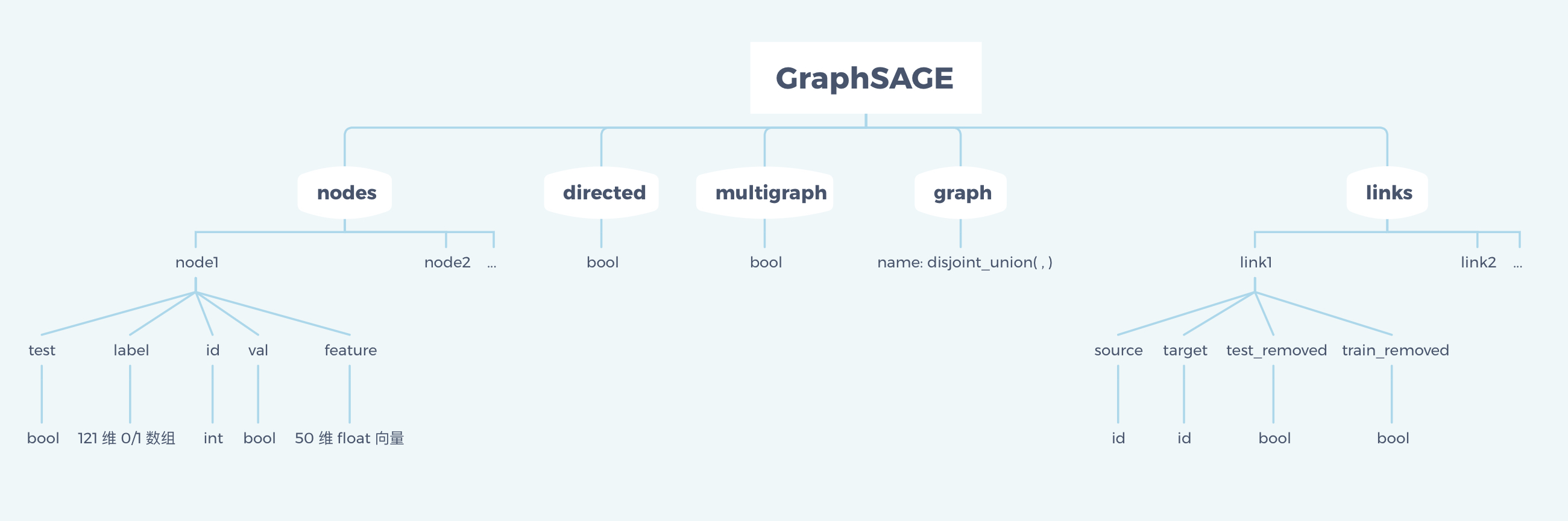展开全文• GraphSAGE源码分析报告 一、 Graphsage简介 0. 什么是深度学习？ 深度学习是一类机器学习算法，它使用多层渐进地从原始输入中提取高级特征。例如，在图像处理中，较低的层可能识别边缘，而较高的层可能识别与人类...


GraphSAGE源码分析报告
一、 Graphsage简介0.什么是深度学习？1.什么是Graphsage？2.Grpaphsage有什么特点？3.怎么使用Grpaphsage算法程序？
二、主要功能分析与建模0. 功能选取1.需求建模（1）WHAT（2）WHY（3）需求分析
2.执行流程
三、类的设计以及关联分析四、高级意图设计分析1.工厂方法模式2.单例模式
五、总结

写在前面：本分析报告是我在中国科学院大学王伟老师的面向对象程序设计大作业——对GraphSAGE进行分析以考察面向对象思想在其中的应用。
因为这是我第一篇CSDN文章，所以文本编辑界面还不太熟练，做出来的文章格式可能不大美观，在日后我也会逐步更新并改进文章格式和内容的。如果报告中有不恰当的地方或者想和我一起探讨算法实现的同学们可以直接联系我！

一、 Graphsage简介
0.什么是深度学习？
深度学习是一类机器学习算法，它使用多层渐进地从原始输入中提取高级特征。例如，在图像处理中，较低的层可能识别边缘，而较高的层可能识别与人类相关的概念，如数字、字母或面孔。（来自维基百科的深度学习定义） 总的来说，深度学习主要涉及三类方法： (1)基于卷积运算的神经网络系统，即卷积神经网络(CNN)。 (2)基于多层神经元的自编码神经网络，包括自编码( Auto encoder)以及近年来受到广泛关注的稀疏编码两类( Sparse Coding)。 (3)以多层自编码神经网络的方式进行预训练，进而结合鉴别信息进一步优化神经网络权值的深度置信网络(DBN)。 我们目前所接触和学习到的大多是基于卷积运算的神经网络。近年来围绕卷积神经网络而开展的研究取得了相当可观的成果，python也有TensorFlow、pytorch、sklearn等库支持神经网络的计算，如此一来代码的撰写则会方便许多，同时也可以保证模型运行的高效性。
1.什么是Graphsage？
GraphSAGE即Graph SAmple and aggreGatE, 类似于传统的图卷积神经网络GCN，它也是一种图的深度学习算法，它的特点在于引入了Inductive和sample这两个特点。GraphSage的出现完成了机器学习从Transductive（直推式学习）到inductive（归纳式学习）的转变，同时还提出了Mean aggregator、Pooling aggregator、LSTM aggregator这三种聚合函数，拥有更强的表达能力。
2.Grpaphsage有什么特点？
（1）以往GCN算法是典型的直推式学习方法，它所学习到的参数很大程度上与图的结构有感，一旦图发生了变化则需要重新学习参数；而GraphSAGE便是采用归纳式学习方法，它学习节点之间的聚合模式，利用结点领域的聚合模型直接学习处新节点的嵌入特征，只要图不发生太大的变化则无需重新学习参数，大大提高了算法的鲁棒性。（2）引入邻居采样，将直推式节点只表示一种局部结构转变为对应多种局部结构的节点归纳表示，可有效防止训练过拟合，增强泛化能力。 （3）通过邻居采样的方式解决了GCN内存爆炸的问题，适用于大规模图。
3.怎么使用Grpaphsage算法程序？
Graphsage源码可以从GitHub上获取，链接：https://github.com/williamleif/GraphSAGE。

Graphsage整体分为有监督学习和无监督学习部分，分别对应supervised_train.py和unsupervised_train.py文件，每个文件中均有自己单独的main函数，在运行的时候只需在对应的文件里运行即可。 具体的API也分为有监督学习和无监督学习的，此处以有监督学习为例。在supervised_train.py文件中，存在main函数：
def main(argv=None):
train(train_data)

def load_data(prefix, normalize=True, load_walks=False):
...
return G, feats, id_map, walks, class_map

根据函数的返回值我们大致可以推断出我们需要准备的数据集的内容了，源码网站为我们提供了一个数据集格式的标准以及数据集示例，如下：此处以toy-ppi数据集为例，其图（G）、class_map以及id_map需要是json格式的文件，feats需要npy型文件（可以调用python的numpy库来生成），walks需要txt型文件。 之后调用train函数并代入load_data函数处理得到的以上五个数据即可完成训练，具体内部的实现逻辑因为不是本课程的重点就不详细叙述了，在接下来的分析中将会重点针对面向对象的思想进行分析而非算法本身。 为了方便用户使用graphsage程序，开发者专门撰写了一个example_supervised.sh文件，里面包含了运行程序需要输入的命令行，在命令行内可以指定聚合的方式等具体实现中的算法。如果需要更改内部算法进行训练则只需更改example_supervised.sh文件中命令行的参数即可，以下是命令行的一个示例：
python -m graphsage.supervised_train --train_prefix ./example_data/ppi --model graphsage_mean --sigmoid

该例子中./example_data/ppi表示数据集的路径，supervised_train表示采用的是有监督学习， graphsage_mean表示采用的聚合方法是Mean aggregator。在linux系统中，设置好了数据集以及命令行参数后，只需输入：
sudo ./example_supervised.sh

二、主要功能分析与建模
0. 功能选取
此处主要针对有监督学习部分的功能进行分析。有监督学习是从标签化训练数据集中推断出函数的机器学习任务，比较像我们平时做题的过程：从已知答案的练习题中推断出做题规律，从而很好地运用在考试上。
1.需求建模
对于机器学习，我们所需要的是利用训练集对模型进行训练，并利用测试集测试模型的准确度。运用需求模型，我们可以得到以下的分析：
（1）WHAT
程序需要根据用户提供的数据集，利用图（G.json文件）中邻居节点的特征，为先前未见过的数据有效地生成节点的 Embedding。
（2）WHY
大图学习容易造成内存溢出以及时间过长等问题，在暂时无法提升硬件水平的情况下，设计出更高效的算法相对来说是更好的选择。
（3）需求分析
采用用例法，包含正常处理、异常处理以及替代处理的流程

【用例名称】 Graphsage有监督学习算法 【场景】 Who：用户、程序、数据集 Where：内存 When：运行时（训练时） 【用例描述】 1.用户将准备好的数据集放到指定的文件目录下 2.用户在命令行参数处设置好数据集路径和聚合方式，并运行该sh文件。 2.1输入路径不存在，或者该路径下无有效数据集，打印报错信息“path error” 2.2命令行输入格式出错，打印报错信息“unknown command” 3.程序解析命令行，并开始运行相关的main函数 4.运行load_data函数，从数据集中读取数据 4.1遇到无效的数据，或者不符合格式的数据，打印报错信息“invalid data” 4.2数据量过大导致电脑内存无法容纳，打印报错信息“unable to allocate memory” 5.运行train函数，开始训练 5.1输入的模型不存在，打印报错信息“Error: model name unrecognized.” 5-A：程序采用graphsage_mean模型进行训练 5-B：程序采用gcn模型进行训练 5-C：程序采用graphsage_seq模型进行训练 5-D：程序采用graphsage_maxpool模型进行训练 5-E：程序采用graphsage_meanpool模型进行训练 6.进行validation，验证训练结果 7.打印训练结果（包括loss、mic、mac、time等信息），用户得到该信息 8.利用训练结果对新节点进行预测 【用例价值】 程序在训练完后用户可以得到训练的Graphsage模型，从而为先前未见过的数据有效地生成节点的 Embedding。 【约束和限制】 1.输入的数据集的格式应规范。 2.用户必须下载程序所需使用的拓展包，并且拓展包的版本也有要求（见requirement.txt文件） 3.程序的运行需要一定的环境，环境的安装方法在Dokerfile文件中有介绍。

我们从中提取出关键的名词和动词

【名词】：用户、程序、模型、validation、数据集、命令行 【动词】：训练、预测、打印、解析

我们可以发现，用户只是业务的参与者，没有明确的用处。同时数据集以及命令行都是用户准备好的一个输入，与程序本身并无太大的关系。Validation只是train函数中的一种方法，用于验证训练结果的，起到的只是一个辅助的作用。因此真正有用的名词就是“程序”。 对于动词来说，打印只需用print函数实现即可，没必要专门算作某一类的功能。解析命令行也是每个程序通用的功能。因此“训练”和“预测”便是关键动词。 因此我们大致抽取出来的类及其方法和属性为：

【类】supervised_models（程序） 【属性】loss、model_size等 【方法】build（训练）、predict（预测）

2.执行流程
简单来分析，graphsage有监督学习的训练逻辑大致如下图：可以观察出图中每个结点都有一个或者多个邻居，图2展示了聚合的过程，蓝色结点将它的邻居（绿色结点）的特征集合在自己身上，红色结点便将其蓝色结点邻居的特征集中在自己身上，通过这样一层一层的聚合便可以计算得出结点的特征，从而用于新节点的预测。更直观的工作过程图如下：它的核心算法是对每一层将每一个点的邻居特征都聚合起来，所以需要使用两层循环来完成这个功能，具体伪代码如下：（如果对具体算法实现不感兴趣可以不用深究）在直观地了解了工作流程后，我们看看它是如何体现在代码上的。首先看看代码框架：程序主要调用的是supervised_train.py文件中的train函数，在train函数中，首先会获得数据集的信息：
   G = train_data
features = train_data
id_map = train_data
class_map  = train_data

然后根据命令行选中的模型，进行不同的处理，此处以graphsage_mean模型为例：
if FLAGS.model == 'graphsage_mean':
# Create model
……
model = SupervisedGraphsage(num_classes, placeholders,
features,
minibatch.deg,
layer_infos,
model_size=FLAGS.model_size,
sigmoid_loss = FLAGS.sigmoid,
identity_dim = FLAGS.identity_dim,
logging=True)

class UniformNeighborSampler(Layer):
super(UniformNeighborSampler, self).__init__(**kwargs)
def _call(self, inputs):
ids, num_samples = inputs

接着创建模型，利用SupervisedGraphsage这一个类创建一个对象model，这个类包含三种方法：build、loss和predict。它正是有监督学习算法的核心类。
class SupervisedGraphsage(models.SampleAndAggregate):
def __init__(self, num_classes,
layer_infos, concat=True, aggregator_type="mean",
model_size="small", sigmoid_loss=False, identity_dim=0,**kwargs):
......
def build(self):
samples1, support_sizes1 = self.sample(self.inputs1, self.layer_infos)
......
self.preds = self.predict()
def _loss(self):
# Weight decay loss
for aggregator in self.aggregators:
for var in aggregator.vars.values():
......
def predict(self):
if self.sigmoid_loss:
return tf.nn.sigmoid(self.node_preds)
else:
return tf.nn.softmax(self.node_preds)

在得到了训练模型和sample之后，便需要初始化相关变量和任务然后开始训练了：
# Initialize session
sess = tf.Session(config=config)
merged = tf.summary.merge_all()
summary_writer = tf.summary.FileWriter(log_dir(), sess.graph)
# Init variables

之后的过程便是涉及到具体的算法了，大致就是建立相应的字典，然后调用训练模型得到训练结果，再进行validation，最后打印出学习到的模型中相应的参数，代码框架如下:
 # Train model
for epoch in range(FLAGS.epochs):
......
while not minibatch.end():
# Construct feed dictionary
......
# Training step
......
if iter % FLAGS.validate_iter == 0:
# Validation
......
# Print results
if total_steps % FLAGS.print_every == 0:
train_f1_mic, train_f1_mac = calc_f1(labels, outs[-1])
print("Iter:", '%04d' % iter,......
......

分析到这里我们发现实际上就有监督学习来说，类的数量还是比较少的，机器学习的重心还是在算法本身上，一般一种学习方法只需要单独设计一个类即可，不需要太多类之间的频繁交互。不过无论使用哪种学习方法，都需要采样，也都需要聚合，所以将必须会使用到sample以及aggregate对应的类。将这两个类封装好可以为程序的设计带来很大的便利。同时以上的几点也体现了模块设计的“高内聚、低耦合”原则，模块之间功能相互独立，不需要互相依赖，一个模块的错误不至于引发严重的连锁反应。
三、类的设计以及关联分析
上一节主要针对算法流程进行分析，这一节就针对类的设计以及其间的关联进行分析。 整体上整个项目文件的UML图为：从图中我们可以发现，整个项目的类虽然不少，但是联系却十分紧密，个别类之间是继承关系，而更多类之间是组合的关系。只有三个类保持与其它类相对独立的关系。组合关系占领首要地位说明设计遵循了组合/聚合复用原则，尽量使用组合/聚合达到复用而非继承，因此代码中更多的是直接复用现有的类来创建对象，而非再用继承关系形成很多子类，使框架更加复杂。 整体上，类之间的关系也体现出了算法的逻辑，比如supervisedGraphsage由MeanAggregator、GCNAggregator、MaxPoolingAggregator、MeanpoolingAggregator以及SeqAggregator组合而成，可以得到实际上有监督学习算法里面包含了五种聚合模式。其次，supervisedGraphsage是继承SampleandAggregate类的，说明有监督学习也是一种先采样然后聚合得到模型的一种算法。 上图中大致存在两大块继承关系： 1.以Layer为父类，它包含了Dense、UniformNeighborSampler、MeanAggregator以及BipartiteEdgePredLayer为子类，整体上来说，Layer是一个基础层类，它为所有的层的对象定义了基本的API，相当于是其它所有类的一种基恩框架。 2.以Model为父类，包含四种方法build、load、predict和save。它存在两个子类MLP和GeneralizedModel，分别只拥有方法predict和build，其中GeneralizedModel是base class，是基础模型；而MLP是一个标准的多层感知器。GeneralizedModel又存在两个子类，分别是Node2VecModel和SampleAndaggregate, SampleAndaggregate是graphsage无监督训练模型的基本实现，而Node2VecModel是一个转换器，是一个将节点转换成向量的模型。最后SampleAndaggregate存在一个子类supervisedGraphsage，为有监督学习的训练和预测模型。 通过对每一个类的功能仔细分析我们可以发现，虽然类之间的关联性比较多，但是每一个类的功能都是相互独立的。因为就机器学习来说，每个类基本上都是为了一种功能而设计的，是按照算法流程来的，比如采样就是采样，采样后再将样本点进行聚合。设计的时候会避免出现边采样边聚合的情况，因为类的设计需要保证单一职责原则，采样与聚合同时进行将会导致模块间的耦合程度过大，违反了“高内聚，低耦合的原则”。 对于有监督训练模型，它的UML图如下：它是models.py文件中SampleAndaggregate类的继承，可以看出它包含build以及predict两种方法，分别对应训练和预测。其内部包含了很多属性，分别是在算法过程中需要使用到的信息，此处就不再详细解释每个变量的作用了。从这里可以看出整个项目的设计还是很有条理性的，因为项目中会使用到很多模型，所以它就在最开始设计了一个总的模型Model（相当是定义了一个模型的规范），然后在构造具体的模型的时候可以选择继承Model来表示是比它更加细化、功能更明确的一个模型。
四、高级意图设计分析
1.工厂方法模式

意图： 定义一个用于创建对象的接口，让子类决定实例化哪一个类。 主要作用： 将类的实例化（具体产品的创建）延迟到工厂类的子类（具体工厂）中完成，即由子类来决定应该实例化（创建）哪一个类。 应用场景：工厂需要频繁生产新产品的时候 如何解决： 采用工厂模式，利用if-else结构，添加新产品或者新算法的时候只需增加一层else的逻辑即可。

工厂方法模式在本项目中有两点典型的体现： （1）第一个是在有监督学习的train函数中，有一系列的if-else结构来针对命令行输入的聚合模型选择生成sample以及model，代码框架如下：
if FLAGS.model == 'graphsage_mean':
......
elif FLAGS.model == 'gcn':
......
elif FLAGS.model == 'graphsage_seq':
......
elif FLAGS.model == 'graphsage_maxpool':
......
elif FLAGS.model == 'graphsage_meanpool':
......
else:
raise Exception('Error: model name unrecognized.')

虽说这不是像java里典型的工厂方法模式，但是存在工厂方法模式的思想，针对不同的聚合方法，没有必要为每一种方法都专门设计一个类，这样就会使得类的数量过于多了，使结构更加复杂。 为了方便用户比对不同算法的运行结果并选取最好的算法建立模型，一般在设计的时候会设计多种聚合方法，尽管这是与GCN不同的graphsage算法，但还是在代码里保留了GCN的算法，为的就是将两种算法得到的结果进行对比。或者从另一个角度可以理解为，产品更新换代的时候并没有完全抛弃旧的版本，而是在旧版本的基础上再添加新版本。 这种设计模式非常方便算法的更新换代，一旦研究出某一种新的聚合方法，只需要添加一个新的else分支，然后再在这个分支下撰写此方法对应的代码即可（因为这些方法的初始化以及训练过程的流程都是一样的）。 （2）第二个是在SupervisedGraphsage类里面：
if aggregator_type == "mean":
self.aggregator_cls = MeanAggregator
elif aggregator_type == "seq":
self.aggregator_cls = SeqAggregator
elif aggregator_type == "meanpool":
self.aggregator_cls = MeanPoolingAggregator
elif aggregator_type == "maxpool":
self.aggregator_cls = MaxPoolingAggregator
elif aggregator_type == "gcn":
self.aggregator_cls = GCNAggregator
else:
raise Exception("Unknown aggregator: ", self.aggregator_cls)

此处相当于是根据创建对象时传入的聚合类型，为该类的aggregator_cls属性设置相应的值。之后再根据这个属性来确定具体需要创建哪一个用于聚合的类（MeanAggregator、GCNAggregator、MeanPoolAggregator、MaxPoolAggregator和SeqAggregator中任选一个）。
2.单例模式

意图：保证一个类仅有一个实例，并提供一个访问它的全局访问点。 主要解决：一个全局使用的类被频繁地创建与销毁。 应用场景：需要控制实例数目、节省系统资源的时候。 如何解决：只创建一个实例，且不销毁。

通过观察整个项目的类图我们可以发现，除了联系紧密的各个类之外，还有两个类是独立于他们之外的，即它们没有继承任何类，也没有组合关系。我们首先来观察他们的功能，EdgeMinibatchIterator是一个小批迭代器，它在一批抽样边或随机对共现边上迭代；NodeMinibatchIterator也是一个迭代器，它在节点上迭代以进行有监督学习。（UML图如下）这两个迭代器的作用是在采样的过程中，分别用于处理图的边和节点信息的类，比如construct_adj方法是建立邻接表的方法，同时也会返回每个顶点的度。在采样的过程中如果顶点邻居不足那就抽样补满128个。 因为针对每一个输入的数据，都需要进行如上的操作，所以不如直接为它提供一个全局访问点，这种方法有点类似java里面的单例模式。因为毕竟python不是java语言，所以表现形式上难免会有不同，但是思想还是一样的。
class EdgeMinibatchIterator(object):
......
class NodeMinibatchIterator(object):
......

这样的模式使得这些类不会被频繁地创建与销毁，当每次处理数据的时候直接使用这两个迭代器即可。
五、总结
整体上我感觉，其实深度学习一类的代码更侧重于算法实现逻辑，类与类之间的关系并没有一些大项目那么明显，而且类的方法基本脱离不开训练与预测这两种。不过话说回来，面向对象思想的应用可以使得模型的设计思路更加清晰，不仅有利于代码撰写，还有利于算法设计。对类进行封装还有利于维护代码的安全性，不至于用户修改到关键代码导致程序崩溃。因此，将一个本可以全部使用函数而实现的深度学习算法划分为一个一个类，并使用相互继承的关系实现整个算法流程是一种成熟的做法。往后对代码进行维护和优化的时候也会更加方便（可以对每一个类单独进行调试）。 其实我对graphsage的算法本身也挺感兴趣的，所以除了前文提到的一些优点之外，我还发现了本算法的一些缺点：
首先它无法处理加权图，而只能从邻居处等权聚合特征，这算是一个比较大的局限性。其次该算法的采样引入了随机过程，推理阶段同一节点embedding特征不稳定，且邻居采样会导致反向传播时会带来较大梯度方差最后，采样数目的限制会导致部分节点的重要局部信息丢失 针对以上三个缺点，我想到一些改进的措施：为了处理加权图，在聚合之前需要将邻居特征归一化，也就是根据这条边的权重相应地修改邻居的特征向量，最后再进行特征融合为了处理embedding特征不稳定的问题，可以对搜索进行剪枝，思路类似于KNN算法。直接对原始网络进行剪枝操作，只保留每个节点权重最大的K条边。提前对每一个节点的特征与其所有邻居特征的均值进行合并，这样就可以使每一个节点初始状态下就拥有周围邻居节点的一些信息，通过该种方式在采样相同节点的前提下可获得更多的局部信息
因为本课程的侧重点不在代码的具体实现上，所以我也只能在总结出多发表一下自己对代码逻辑的看法。不过面向对象程序设计这门课使我意识到，有时候一个完整而合理的框架甚至比代码逻辑本身更加重要，尤其是进行大项目设计的时候。本学期进行完计算机体系结构实验课后这一点我深有体会，当代码量大的时候，代码直接的逻辑的清楚性显得尤为重要，一旦代码框架没有组织好，调bug的时候将会无从下手，十分痛苦。如果说设计一个项目是建一所楼房的话，那么面向对象思想架构就是地基，它有多牢固、多可靠决定了楼房能建多高。
展开全文算法
• pytorch geometric教程三 GraphSAGE代码详解+实战pytorch geometric教程三 GraphSAGE代码详解&实战原理回顾paper公式代码实现SAGE代码（SAGEConv）__init__邻域聚合方式参数含义 pytorch geometric教程三 ...


pytorch geometric教程三 GraphSAGE源码详解+实战
pytorch geometric教程三 GraphSAGE源码详解&实战原理回顾paper公式代码实现
SAGE代码（SAGEConv）__init__邻域聚合方式参数含义init主体
forward函数参数forward主体
消息传递一，edge_index为Tensor二，edge_index为SparseTensor

实战定义模型模型调用

pytorch geometric教程三 GraphSAGE源码详解&实战
这一篇是建立在你已经对pytorch geometric消息传递&跟新的原理有一定了解的基础上。如果没有的话，也没关系，可以看看这篇关于pytorch geometric消息传递&更新的博文（pytorch geometric 消息传递源码详解（MESSAGE PASSING）+实例）。 GraphSAGE图算法中的SAGE是sample and aggregate的缩写。这一篇博文讲的是SAGEConv代码中实现的卷积部分，也就是SAGE中aggregate部分。sample的部分在pytorch geometric中是在torch_geometric.loader.NeighborSampler实现的(老一点的版本是在torch_geometric.data.NeighborSampler)。sample方法极大地增加了图算法的可拓展性，实现了minibatch跟新梯度，使得图的大规模计算成为可能。sample部分指路这篇博文（pytorch geometric教程四 利用NeighorSampler实现节点维度的mini-batch + GraphSAGE样例）。下文提到的SAGE都默认只有aggregate部分。
原理回顾
先回顾一下SAGE的原理：
paper公式

x

i

′

=

W

⋅

c

o

n

c

a

t

(

A

g

g

r

e

g

a

t

e

j

∈

N

(

i

)

x

j

,

x

i

)

\mathbf{x}^{\prime}_i = \mathbf{W} \cdot concat( \mathrm{Aggregate}_{j \in \mathcal{N(i)}} \mathbf{x}_j, \mathbf{x}_i)

其中点

j

j

是点

i

i

的邻居。直观理解，对邻居特征进行某种方式聚合后，和节点自身特征concat，再进行一个维度变换。其中聚合方式有mean, Pooling, LSTM。
代码实现

x

i

′

=

W

1

x

i

+

W

2

⋅

A

g

g

r

e

g

a

t

e

j

∈

N

(

i

)

x

j

\mathbf{x}^{\prime}_i = \mathbf{W}_1 \mathbf{x}_i + \mathbf{W}_2 \cdot \mathrm{Aggregate}_{j \in \mathcal{N(i)}} \mathbf{x}_j

这是pytorch geometric实现的方式。注意到代码实现中，concat消失了，

W

1

\mathbf{W}_1

，

W

2

\mathbf{W}_2

取代了

W

\mathbf{W}

。其实这两个结果是一样的。可以看一下示意图：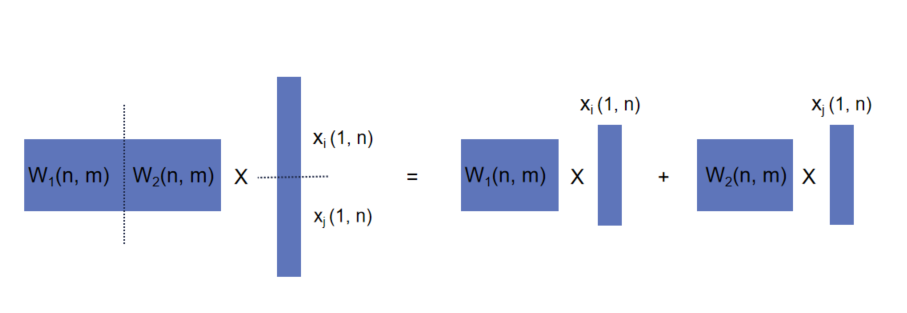这里直观理解SAGE，将邻居对应的特征聚合后，进行一个维度变换，再加上节点自身经过维度变换的特征，就是节点最终生成的embedding。相比GCN，节点

i

i

和邻居

j

j

使用了不同的

W

\mathbf{W}

，投射到了不同的特征空间，这一点大大加强了模型的表达能力。mean是代码中默认的邻居聚合方式，还可以改成max, add。原paper中的Pooling, LSTM聚合方式，SAGEConv是没有实现的。 再次强调一下，SAGEConv代码中的邻居就是你传入的邻居，不管是使用NeighborSampler等方式对邻居进行采样过的邻居还是未采样的所有邻居，它只管接收你传入的邻居，邻居采样不在这里实现。
SAGE代码（SAGEConv）
init
class SAGEConv(MessagePassing):
def __init__(self, in_channels: Union[int, Tuple[int, int]],
out_channels: int, normalize: bool = False,
root_weight: bool = True,
bias: bool = True, **kwargs):  # yapf: disable
kwargs.setdefault('aggr', 'mean')
super(SAGEConv, self).__init__(**kwargs)

self.in_channels = in_channels
self.out_channels = out_channels
self.normalize = normalize
self.root_weight = root_weight

if isinstance(in_channels, int):
in_channels = (in_channels, in_channels)

self.lin_l = Linear(in_channels, out_channels, bias=bias)
if self.root_weight:
self.lin_r = Linear(in_channels, out_channels, bias=False)

self.reset_parameters()

邻域聚合方式
kwargs.setdefault('aggr', 'mean')检查关键字参数中是否定义了邻域聚合方式，也就是是否包含名为aggr的key。如果没有的话，采用默认的mean聚合方式，也就是邻居特征求和平均。在我们定义model的时候，我们可以通过参数aggr = add, mean, max来选择邻居特征聚合方式。
参数含义
另外解释下各个参数的含义：
in_channels: Union[int, Tuple[int, int]]：输入原始特征或者隐含层embedding的维度。如果是-1，则根据传入的x来推断特征维度。注意in_channels可以是一个整数，也可以是两个整数组成的tuple，分别对应source节点和target节点的特征维度，也是参数

W

2

\mathbf{W}_2

和

W

1

\mathbf{W}_1

的shape。out_channels：输出embedding的维度normalize：默认是False, 如果是True的话，对output进行

ℓ

2

\ell_2

归一化，

x

i

′

∥

x

i

′

∥

2

\frac{\mathbf{x}^{\prime}_i} {\| \mathbf{x}^{\prime}_i \|_2}

improved: 默认是False, 如果是True的话，则

A

^

=

A

+

2

I

\mathbf{\hat{A}} = \mathbf{A} + 2\mathbf{I}

，增强了自身的权重。root_weight: 默认是True。如果是False的话，output不会加上节点自身特征转换维度后的值，就是代码实现公式中的

W

1

x

i

\mathbf{W}_1 \mathbf{x}_i

。bias：默认是True，如果是False的话，layer中没有bias项。
init主体
init函数包括了

W

1

\mathbf{W}_1

和

W

2

\mathbf{W}_2

的定义。
forward函数
	def forward(self, x: Union[Tensor, OptPairTensor], edge_index: Adj,
size: Size = None) -> Tensor:
""""""
if isinstance(x, Tensor):
x: OptPairTensor = (x, x)

# propagate_type: (x: OptPairTensor)
out = self.propagate(edge_index, x=x, size=size)
out = self.lin_l(out)

x_r = x
if self.root_weight and x_r is not None:
out += self.lin_r(x_r)

if self.normalize:
out = F.normalize(out, p=2., dim=-1)

return out

参数
x：Union[Tensor, OptPairTensor]：可以是Tensor，也可以是OptPairTensor (pyg定义的tuple of Tensor)。 当图是bipartite的时候，x是OptPairTensor ，source节点特征对应x，在代码中赋值给x_l变量，target节点特征对应x，在代码中赋值给 x_r。这也是作者把

W

1

\mathbf{W}_1

定义为lin_r，

W

2

\mathbf{W}_2

定义为lin_l的原因。 另外pyg中实现sample是通过NeighborSampler返回bipartite子图完成的。所以SAGEConv支持邻居采样的结果，与NeighborSampler一起使用，可以实现minibatch训练大规模图数据。edge_index: Adj: Adj是pyg定义的邻接矩阵类型，可以是Tensor，也可以是SparseTensor。
forward主体
代码的逻辑是比较清晰的：
如果x是Tensor的话，将x: OptPairTensor = (x, x) 为了兼容同构图与bipartite图，将x写成bipartite图的形式。而在同构图中，source节点和target节点对应的x是一样的，所以有(x, x)。调用propagate函数对进行消息传递和聚合，输出的out对应公式中的

A

g

g

r

e

g

a

t

e

j

∈

N

(

i

)

x

j

\mathrm{Aggregate}_{j \in \mathcal{N(i)}} \mathbf{x}_j

。对progagate函数底层逻辑有疑问的小伙伴，可以参考之前的博文。self.lin_l(out)对应

W

2

o

u

t

\mathbf{W}_2 \, \mathbf{out}

self.lin_r(x_r)对应

W

1

X

\mathbf{W}_1 \, \mathbf{X}

消息传递
    def message(self, x_j: Tensor) -> Tensor:
return x_j

x: OptPairTensor) -> Tensor:

一，edge_index为Tensor
这里不明白的小伙伴可以先看这篇博文（pytorch geometric 消息传递原理详解（MESSAGE PASSING）+实例） edge_index为Tensor的时候，propagate调用message和aggregate实现消息传递和更新。 这里message函数对邻居特征没有任何处理，只是进行了传递，所以最终propagate函数只是对邻居特征进行了aggregate。
二，edge_index为SparseTensor
edge_index为SparseTensor的时候，propagate函数会在message_and_aggregate被定义的情况下被优先调用，代替message和aggregate。 这里message_and_aggregate直接调用类似矩阵计算matmul(adj_t, x, reduce=self.aggr)。x是source节点的特征。matmul来自于torch_sparse，除了类似常规的矩阵相乘外，还给出了可选的reduce，所以除了add，mean和max也是可以在这里实现的。
实战
定义模型
pytorch geometric的卷积层调用还是挺简单的，下面是一个两层的SAGE。
import torch
import torch.nn.functional as F
from torch_geometric.nn.conv import SAGEConv

class SAGE(torch.nn.Module):
def __init__(self, in_channels, hidden_channels, out_channels, dropout=0.):
super(SAGE, self).__init__()

self.convs = torch.nn.ModuleList()
self.convs.append(SAGEConv(in_channels, hidden_channels))
self.convs.append(SAGEConv(hidden_channels, out_channels))

self.dropout = dropout

def reset_parameters():
for conv in self.convs:
conv.reset_parameters()

def forward(self, x, edge_index):
x = self.convs(x, edge_index)
x = F.relu(x)
x = F.dropout(x, p=self.dropout, training=self.training)
x = self.convs(x, edge_index)

return x.log_softmax(dim=-1)

模型调用
接下来，我们用Cora数据集尝试一下。
#读取数据
from torch_geometric.datasets import Planetoid
import torch_geometric.transforms as T

transform = T.ToSparseTensor()
dataset = Planetoid(name='Cora', root=r'./dataset/Cora', transform=transform)
data = dataset

model = SAGE(in_channels=dataset.num_features, hidden_channels=128, out_channels=dataset.num_classes)

def train():
model.train()

loss.backward()
optimizer.step()

return loss.item()

def test():
model.eval()

y_pred = out.argmax(axis=-1)

correct = y_pred == data.y

return train_acc, valid_acc, test_acc

#跑10个epoch看一下模型效果
for epoch in range(20):
loss = train()
train_acc, valid_acc, test_acc = test()
print(f'Epoch: {epoch:02d}, '
f'Loss: {loss:.4f}, '
f'Train_acc: {100 * train_acc:.3f}%, '
f'Valid_acc: {100 * valid_acc:.3f}% '
f'Test_acc: {100 * test_acc:.3f}%')

Epoch: 00, Loss: 1.9485, Train_acc: 67.143%, Valid_acc: 39.400% Test_acc: 37.200%
Epoch: 01, Loss: 1.8761, Train_acc: 90.714%, Valid_acc: 51.400% Test_acc: 51.700%
Epoch: 02, Loss: 1.8071, Train_acc: 99.286%, Valid_acc: 59.600% Test_acc: 60.800%
Epoch: 03, Loss: 1.7365, Train_acc: 99.286%, Valid_acc: 66.200% Test_acc: 67.100%
Epoch: 04, Loss: 1.6615, Train_acc: 100.000%, Valid_acc: 70.000% Test_acc: 70.900%
Epoch: 05, Loss: 1.5816, Train_acc: 100.000%, Valid_acc: 73.800% Test_acc: 73.700%
Epoch: 06, Loss: 1.4967, Train_acc: 100.000%, Valid_acc: 76.800% Test_acc: 74.900%
Epoch: 07, Loss: 1.4075, Train_acc: 100.000%, Valid_acc: 77.600% Test_acc: 76.400%
Epoch: 08, Loss: 1.3151, Train_acc: 100.000%, Valid_acc: 77.400% Test_acc: 76.700%
Epoch: 09, Loss: 1.2208, Train_acc: 100.000%, Valid_acc: 77.800% Test_acc: 77.100%
Epoch: 10, Loss: 1.1255, Train_acc: 100.000%, Valid_acc: 77.800% Test_acc: 77.400%
Epoch: 11, Loss: 1.0306, Train_acc: 100.000%, Valid_acc: 78.000% Test_acc: 78.000%
Epoch: 12, Loss: 0.9371, Train_acc: 100.000%, Valid_acc: 78.000% Test_acc: 77.700%
Epoch: 13, Loss: 0.8464, Train_acc: 100.000%, Valid_acc: 78.200% Test_acc: 77.500%
Epoch: 14, Loss: 0.7591, Train_acc: 100.000%, Valid_acc: 77.800% Test_acc: 77.600%
Epoch: 15, Loss: 0.6764, Train_acc: 100.000%, Valid_acc: 77.800% Test_acc: 77.600%
Epoch: 16, Loss: 0.5990, Train_acc: 100.000%, Valid_acc: 77.800% Test_acc: 77.400%
Epoch: 17, Loss: 0.5273, Train_acc: 100.000%, Valid_acc: 77.400% Test_acc: 77.400%
Epoch: 18, Loss: 0.4617, Train_acc: 100.000%, Valid_acc: 77.400% Test_acc: 77.000%
Epoch: 19, Loss: 0.4024, Train_acc: 100.000%, Valid_acc: 77.200% Test_acc: 77.100%

这样我们一个GraphSAGE模型就初步完成啦！我们看到，在经过10个epoch后，test集的acc最高达到了78.0%。对比上篇的GCN代码，可以看到改动只是把卷积从GCNConv换到了SAGEConv，所以pytorch geometric用起来还是很方便的。 欢迎评论交流，转载请注明出处哦！
展开全文pytorch 人工智能 python
• GraphSAGE源码中提供了两种训练方式的入口，supervised_train.py和unsupervised_train.py两种方式，本文只介绍有监督部分，本文从supervised_train.py开始逐步介绍GraphSAGE的思想，旨在讲懂代码中比较繁琐较难...深度学习 神经网络 tensorflow
• GraphSage: Representation Learning on Large Graphs Authors: William L. Hamilton (wleif@stanford.edu), Rex Ying (rexying@stanford.edu) Project Website Alternative reference PyTorch implementation ...
• 知乎专栏：图神经网络 第五篇：个人认为是最详细的一版源码解析 https://zhuanlan.zhihu.com/p/354831060图神经网络 GNN...